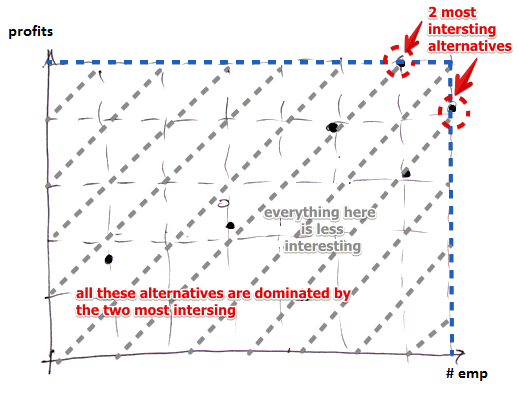# ML Wiki

## Multi-Objective Knapsack Problem

This is a Multi-Objective Optimization problem: a variation of uni-objective Knapsack Problem: In this case instead of maximizing profits we look at multiple objectives.

## Project Selection Problem

We want to select projects for investing some money

• the budget is 900k euros (this this the constraint)

Objectives:

• Maximize expected profits
• Maximize the number of employees
Projects $\to$ $P_1$ $P_2$ $P_3$ $P_4$
Investment, k-euro 200 300 400 500
# of employees 2 3 5 6
Exp. return, % 2.5 4.5 4 2.5

For example

• $P_3 + P_4 \leqslant 900$, can employ 11 peopleWe see that there are only two interesting solutions

• all other solutions are dominated by these two

## Sources

Machine Learning Bookcamp: Learn machine learning by doing projects. Get 40% off with code "grigorevpc".